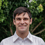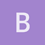This is an archived static version of the original phylobabble.org discussion site.

# Balanced minimum evolution computation in Rematsen

Hello friends–

I’m wondering if there is some way to calculate the BME score in an existing R package. By this score, I mean l in the sense of

There are heaps of functions to infer trees using distance matrices, but here we have a distance matrix and a tree and want to get a number.

@klaus_schliep?

Thanks!

Erickklaus_schliep

Hi @ematsen, if your tree is binary this is trivial, as all the necessary functions exist:

library(ape)
library(phangorn)
library(magrittr)

# a little function for you
get_l <- function(tree, dm){
# make sure the w and d will be in the same order
label <- tree\$tip.label
dm <- as.matrix(dm)[label, label]
dm <- dm[lower.tri(dm)]
tmp <- designTree(tree)
w <- 2^(rowSums(tmp)-2)
sum(dm / w)
}

# a little example
data(yeast)
dm <- dist.ml(yeast)

fastme_tree <- fastme.bal(dm)
get_l(fastme_tree, dm)

# compare with brute force
trees <- allTrees(8, tip.label = names(yeast)) # compute all possible trees
trees %<>% .uncompressTipLabel() %>% unclass   # this speeds up things a bit

bf_l <- sapply(trees, get_l, dm=dm)
bf_tree <- trees[[which.min(bf_l)]]
RF.dist(bf_tree, fastme_tree)


I have no code for the general case with multifurcations (yet). Hope this gets you started.

Cheers, Klausematsen

Very slick! Thanks @klaus_schliep! This will do just fine for us.